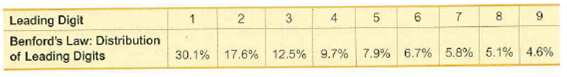# 6.Leading DigitBenford's Law: Distribution9.7% 7.9% 6.7% 5.8% 5.1% 4.6%17.6% 12.5%30.1%of Leading Digits002.

Question
4 views

Author’s Computer Files The author recorded the leading digits of the sizes of the electronic document files for the current edition of this book. The leading digits have frequencies of 55, 25, 17, 24, 18, 12, 12, 3, and 4 (corresponding to the leading digits of 1, 2, 3, 4, 5, 6, 7, 8, and 9, respectively). Using a 0.05 significance level, test for goodness-of-fit with Benford’s law.help_outlineImage Transcriptionclose6. Leading Digit Benford's Law: Distribution 9.7% 7.9% 6.7% 5.8% 5.1% 4.6% 17.6% 12.5% 30.1% of Leading Digits 00 2. fullscreen
check_circle

Step 1

The claim is to test whether the leading digits of the sizes for the document files that have a frequency of occurrences, which follows Benford’s law.

If the claim is false, then the frequencies of leading digits are not taken from a population distribution that follows Benford’s law.

Step 2

Methods for finding the expected frequency:

Method 1: If all the expected frequencies are equal, then E=n/k

Method 2: If the expected frequencies are not equal, then E=np for each category.

Where, n is the total number of frequencies, k is the categories and p is the probability of occurrence.

Here, the total number of frequencies is 511 and there are 9 outcomes, then k is 9. Also, the probabilities are different. Thus, by using the second method, the expected frequencies are calculated as follows.

Substitute n as 170.

Step 3

### Want to see the full answer?

See Solution

#### Want to see this answer and more?

Solutions are written by subject experts who are available 24/7. Questions are typically answered within 1 hour.*

See Solution
*Response times may vary by subject and question.
Tagged in

### Hypothesis Testing